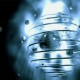# The Difference Between Math and PhysicsRecent headlines boast that string theory is finally testable.  If true, this would mark a significant turning point for the speculative theory.  Touted as a potential TOE, or theory of everything, string theory attempts to unite general relativity (our best theory of the very large) and quantum mechanics (our best theory of the very small).  The theory has been around for decades, but has yet to make a single, testable prediction.  The problem lies in the fact that string theory has so many independent constants, it can accommodate almost any empirical data.

Until now, argues Michael Duff of Imperial College, London.  According to a PhysOrg report, the insight came when Duff attended a “conference in Tasmania where a colleague was presenting the mathematical formulae that describe quantum entanglement: ‘I suddenly recognised his formulae as similar to some I had developed a few years earlier while using string theory to describe black holes. When I returned to the UK I checked my notebooks and confirmed that the maths from these very different areas was indeed identical.'”  His colleague was using the same equations to predict quantum entanglement between 2 and 3 qubits that Duff was using to predict the behavior of black holes.  This led Duff, quite reasonably, to hypothesize that his black-hole equations could be used to make predictions in the more difficult case of quantum entanglement between 4 qubits.  Duff went on to claim that if the equations correctly describe such entanglement relations, then string theory would “work.”

Duff’s mistake was to think that such a calculation would be a test of string theory.  String theory is not just a mathematical equation, it is a theory about the fundamental nature of the universe.  It claims, among other things, that everything in the universe is ultimately made up of loops of strings, vibrating at different frequencies.  Even if the equations of string theory appear in other areas of physics, it does not lend any support to string theory.

To see this, consider the wave equation, which successfully predicts all different kinds of phenomena, ranging from sound waves traveling through the air, to masses oscillating on springs, to self-propagating light waves.  Suppose a scientist developed a theory of light.  In addition to using the wave equation, the theory would include postulates such as: light is a self-propagating wave with electric and magnetic components.  Now, if this scientist attempted to use the wave equation to predict the behavior of sound, it would be a mistake to conclude that this constituted a test of the original theory of light.  If the equations work for sound waves, then that is a convenient mathematical curiosity.  Conversely, if the equations don’t work for sound waves, the theory of light is not cast into doubt.  That is because the theory of light is not broad enough to make any claims about the behavior of sound waves.  Since string theory makes no direct claims about the behavior of 4 entangled qubits, it is fortunate that its mathematical results can be applied in another field, but those results do not tell for or against the theory.

I agree with the comments made by mathematician Peter Woit of Columbia University.  According to Wired, Woit says, “Honestly, I think this is completely outrageous.”  Woit thinks that even if the math is the same, testing the quantum entangled system would only tell you how well you understand the math. “The fact that the same mathematical structure appears in a quantum mechanical problem and some model of black holes isn’t even slightly surprising,” he said. “It doesn’t mean that one is a test of the other.”

Related Articles:

#### Two Dogmas of Neo-Empiricism

By Edouard Machery, University of Pittsburgh
(Vol. 1, June 2006)
Philosophy Compass

Falsifiability

Logic, philosophy of science Popper’s term, also called testability or refutability, for the property …
From The Blackwell Dictionary of Western Philosophy

## 2 thoughts on “The Difference Between Math and Physics”

1.Shahidur Rahman Sikder says:

Albert Einstein’s theory of special relativity formula assimilation E=Mc2 “yet” as I see it Einstein big blunder through that correct assimilation is: E=Mw2 i.e. w= wave. That means this generalized equation is written is E=Mw2, where w represents the speed of the phenomena (e.g., wave) under observation. Since in Einstein’s equation c represents the speed of light, I’m simply replacing w with c to make it specific to that type of phenomena.

2.e1saman says:

I wouldn’t believe it that some professional physicist can make such a mistake. Is the distnace between string theory and reality so huge? 😦